# Analysis of Beams | Shear Force & Bending Moment Diagram Notes pdf ppt Engineering Mechanics

## Analysis of Beams | Shear Force & Bending Moment Diagram:

Beams are structural members, which are most commonly used in buildings. Beams have numerous other applications in case of bridges, automobiles or in mechanical systems. In this article we will see how we can do strength analysis of a beam.

## What is a Beam ?

In a beam transverse load is acted, which in fact comes from the slabs to the column or walls. It is clear from following figure that, beams are integral part of of building structure. In all of the beams load acted is transverse, as shown.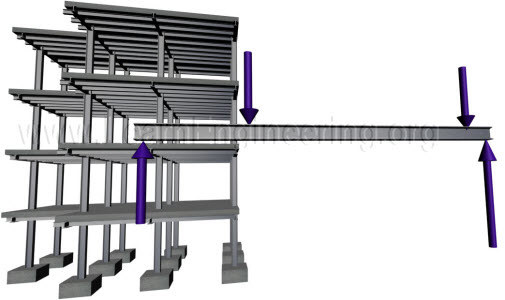Fig.1 In beam transverse load is acted, and it is an integral part of building structure

For analysis purpose, a beam can be considered as a part of the beam column system. This way we can determine external load acting on individual beams. After determining load acting on individual beam, beam can be separated out from beam column system for further analysis.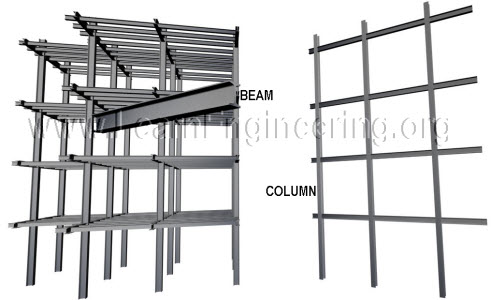Fig.2 Building structure consists of many beam-column systems, beam is a part of beam-column system

Length of the beam is much higher than its lateral dimensions. So axial strain developed in a beam will be very small compared to shear strain, or strain induced due to bending.This is shown in figure below.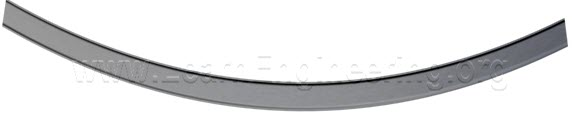Fig.3 Axial strain in beam is negligible compared to shear strain

So for design purpose of beams, analysis of shear force and bending moment induced are of the at most importance. The interesting thing is that you can draw shear force and bending moment distribution along any beam, by understanding what exactly is shear force and bending moment.

Both shear force and bending moment are induced in beam in order to balance external load acting on it. We will go through details of it separately.

## Shear Force:

Shear force is the internal resistance created in beam cross sections, in order to balance transverse external load acting on beam. Consider following beam, it does not matter from where you take a section, when you add forces acting on it, it should be in equilibrium. Shear force is induced exactly for this purpose, to bring the section to equilibrium in vertical direction. It acts parallel to cross section.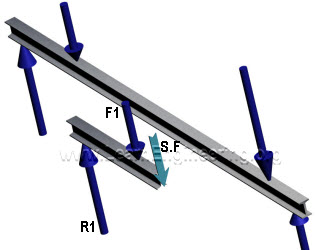Fig.4 Shear force is induced in a section to balance the external load

So just by applying force balance in vertical direction on the free body diagram, we can determine value of shear force at a particular cross section. Usual sign convention of the shear force is as follows.

Now we can apply same concept in different cross section and find out how shear force varies along the length of the beam.

## Bending Moment:

But balance of transverse forces alone does not guarantee equilibrium of a section. There is another possibility of beam rotation, if moment acting on it is not balanced. If this is the case a bending moment will be induced in cross section of beam, to arrest this rotation. It will be induced as normal forces acting on fiber cross section as shown.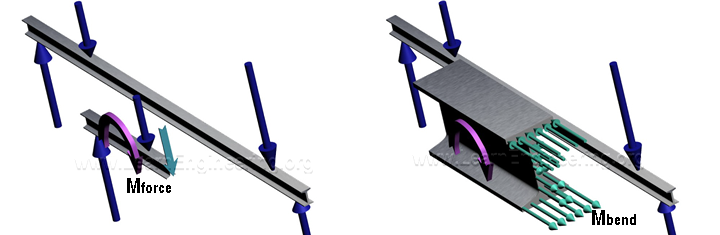Fig.6 Bending moment is induced in section to balance external moment, section is zoomed in left figure for better viewing

Resultant of those forces will be zero, but it will produce a moment, to counter balance the external moment. So we can calculate moment induced at any cross section by balancing the external moment acting on the free body diagram.

Sign convention of bending moment is as follows.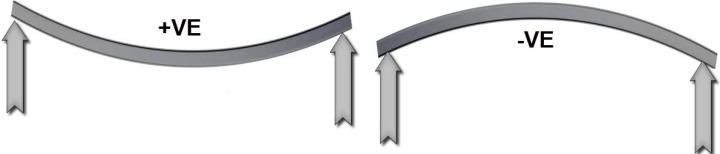Fig.7 Sign convention of bending moment for simply supported case

This sign convention approach is valid for simply supported beam. For cantilever case sign convention is exactly opposite to this.

With these concepts developed, we can easily calculate distribution of shear force and bending moment along the length of the beam. We will see few examples.

## Example of Cantilever:

Consider this case, a cantilever carrying 3 loads.

Here we can start analysis from the free end.

## Section A-B

So for between A and B, if you take a section the only external force acting on it is F1. So a shear force should induce in section to balance this force. So value of shear force between A and B is F1. But force balance alone does not guarantee equilibrium of the section. There is an external moment on the section. So a bending moment will be induced in section, in order to balance the external moment. Since value of external moment is F into x, bending moment will vary linearly.

## Section B-C

Between B and C effect of force F2 also comes. So shear force becomes, F1 plus F2. And in bending moment effect of F2also gets added. Similar analysis is done between section C and D also. So SFD and BMD of this problem would look like this.

## Simply Supported Case:

Now consider this problem. A simply supported beam with uniformly distributed load. First step here would be determination of reaction forces. Since the problem is symmetrical reaction forces will be equal, and will be half of total load acting on beam.

## Section A-B

Lets start analysis from point A. If you take section between point A and B, it should be in equilibrium. So shear force will have equal magnitude of Reaction force. Bending moment gives a linear variation.

## Section B-C

But after point B, effect of point load and distributed load come. Effect of distributed load is something interesting. Take a section in BC. In this section, along with two point loads there is a distributed load also. This distributed load can be assumed as a point load passing through centroid of distributed load. Value of point load is U( x – L/3). And it is at a distance (x – L/3)/ 2 from section line. So shear force will have one more term, which comes from distributed load. From the equation its clear that shear force varies linearly.

You can easily predict, how bending moment varies along length, from the same force diagrams. Since this equation is quadratic it will have a parabolic shape. Same procedure is repeated in remaining section. Since this problem is symmetrical in nature, S.F.D and B.M.D would also be symmetrical. It is shown in figure below.